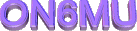By Guy, de ON6MU
rev1.2

A 50 Ohm dummyload is an essential part for any radioamateur as is a powermeter. The prices of such relative simple equipment is expensive, but not for us handy Hams HI. All you need is a metal box (or plastic box painted inside with graphite or other conducting/RF-shielding capable material), a few resistors and basic components (which can be salvaged from old radio's, switching power supplies etc..) and a analog meter. I used a Radio-shack meter, but any (sensitive) meter can be used. It's all a matter of calibrating your meter correctly, which is easy if you can lend a good commercial RF power meter.

This RF-power meter combined with build-in dummy load is made to measure power levels starting from a few milliwatt up to 50 watts (or more if suitable components are used and an avalanche sinterglass diode). IT has 3 scale readings: 0.5 watt, 5 watt and 50 watts. Again, you can extend the scales easily. The power meter is ideal for measuring QRP levels and by adding an BNC connector you'll have an easy oscilloscope measuring point.

In short, an easy and cheap project to build yourself. Even a beginner in HAM homebrewing can make his own fair (if not better then most you buy in the shop) power meter!

Calibration

Is done with a good (commercial or already calibrated) SWR/RF powermeter capable of measuring HF power levels from 5 (or less) to 50 watts and has a frequency range that covers the entire HF-band. You also need a transceiver which you set in series with the meter: TRX -> COMMERCIAL RF METER -> ON6MU RF METER. Set all potentiometers (R2,R3,R4) to maximum resistance. Choose one of the scales (0.5, 5 or 50 watts) to start with. Other power levels/scales with the same step (X1 X10 X100) will have the same indication multiplied. So if you choose scale 2 being 5 watt and calibrate at least 5 power levels of your transceiver it should be ok for the other scale selections. Set R3 for full scale at 5 watt and work your way down. One calibration for all power level settings respectively is sufficient.

You can of course use an oscilloscope or RF voltmeter to calibrate the RF powermeter.
online: https://www.pasternack.com/t-calculator-power-conv.aspx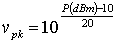volt
Examples:
1W = 30dBm = 10V = 20Vpp)
100mW = 20dBm = 3.16V = 6324mVpp
50mW = 16.99dBm = 2.23V = 4472 mVpp
5mW = 6,99dBm = 0,7V = 1,4mVpp
U = Uv *2 = SQR(Uv)

Schematic fig1Parts list

• alu box (or plastic box painted inside with graphite) of 100mm X 100mm X 50mm

• 1 female PL 259 chassis (SO239)

• 1 connector to be used as oscilloscope measuring point

• Analog Meter (as sensitive as possible and calibrate the scale with a good powermeter)

• C1,C3 = 330pF

• C2,C4 = 47nF high quality

• C5 = 100nF

• C6 = 10uF/6v tantal

• D1,D2 = BYW54...BYW56 (up to 500watt measurements) rev1.2. I used a BYW55 controled avalance rectifier
..........= or 1N1448 if you do not need to measure more than 10 watt max

• D4 = 1N1004 (protects the meter for voltages higher then 0.6 volts)

• S1 = 3 pos. switch (or more if you want more power scales)

• L Dr = 500uH or 1M Ohm carbon resistor 1 watt covered with 0,2mm Cul 3 times (or more) turned over the length of the resistor

• R1 = 18k

• R2 = 100k variable resistor

• R3 = 250k variable resistor

• R4 = 1M variable resistor

• R5 = 47...4k7 (optional; ideal should be configured as an attenuator if you add a connector to be used for measuring the RF with an oscilloscope, RF voltmeter, spectrum analyser...)

• Rd = 50 Ohm Dummy load of at least 20 watt (see text)*

Specifications

• precision power meter capable of measuring power scales of 500mW...100W+ (depending on the dummy load)

• frequency range: entire HF band 100kHz...150Mc

• switchable scale ranges (in this schematic 0.5W, 5W, 50Watts...)

• oscilloscope measuring point

• can be used as dummy load also HI

Note rev1.2*: sometimes the diodes broke when measuring 50watts. To measure power levels up to 100 watts or more a better diode (BYW55) has been used that is suitable for high voltages (Standard Avalanche Sinterglass Diode).

Inside the powermeter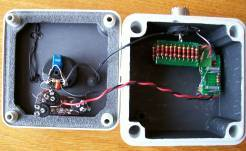Is build out of 20 carbon resistors (or non inductive metalfilm) of 1K and 1Watt all parallel. I used two 15mm X 50mm print boards and soldered two times 10 resistors on each side. Solder the two parts on top of each other. See fig1 and 2. Do not use inductive type of resistors! Always use carbon based resistors or non inductive metalfilm ones. This dummy load is able to dissipate 21 watts continues and no problem to handle a 10 second peek of 50 watts. Long enough to measure the power. Be sure not to transmit high power > 21 watt for a long time as this will burn out your dummy load! IF you need the dummy load to handle more power then you could use 45 2k2 1 watt resistors which doubles the amount of power (and peek power). Of course you can use 1 k resistors of a higher power rating as long as they are non-inductive resistors.

fig.2.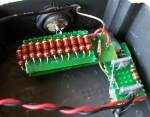2nd example:
RE-M3RFP Microwatt RF Powermeter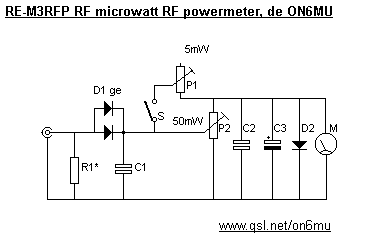Parts list

• shielded box

• a BNC connector

• D1 = 2 x ge diodes, like 1N34, 1N60, 1N270 or equivalents

• C1 = 22nF

• C2 = 100nF

• C3 = 10uF

• D2 = any si diode

• S = switch that toggles betseen 50mW and 5mW (or any other scale you wanted). Switch Open = highest rating, in this case 50mW

• M = a sensitive uV meter

• R1 = 0,5 watt 47 Ohm non inductive resistor

• P1 = 2k5 trimmer

• P2 = 5k trimmer

• Don't forget to check these out:

• .ON6MU Homebrew projects